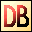DelphiBasicsRandomFunction Generate a random floating point or integer number System unit
 1 function Random : Extended; 2 function Random ( LimitPlusOne : Integer ) : Integer;
Description
The Random function generates random numbers. They can be floating point numbers in the range :

0 <= Number < 1.0

or integer numbers in the range :

0 <= Number < LimitPlusOne

Delphi uses a pseudo random number generator that always returns the same sequence of values (232) each time the program runs.

To avoid this predictability, use the Randomize procedure. It repositions into this random number sequence using the time of day as a pseudo random seed.
Related commands
 Randomize Reposition the Random number generator next value RandomRange Generate a random integer number within a supplied range RandSeed Reposition the Random number generator next valueDownload this web site as a Windows program.